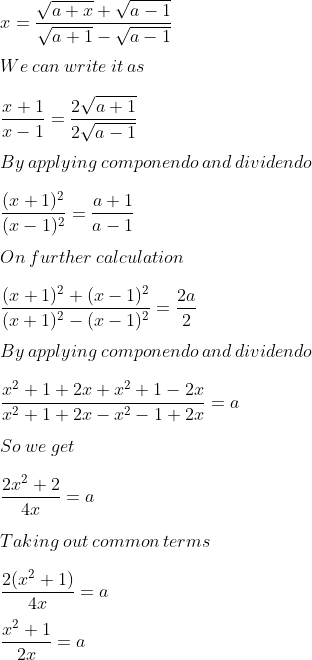Guru

# If x=root(a+x) +root(a−1) /root(a+1) −root(a−1) , using properties of proportion , show that x² – 2ax + 1 = 0

• 0

exam oriented an important question from ML aggarwal, class 10th, chapter 7, ratio and proportion, Avichal publicatio

This question has been asked in previous year question paper 2012.

We have given the value of x and we have to prove the given equation true by putting this value of x.

Ques 14, 7.3

Share

1. Solution:

It is given thatWe get

2ax = x2 + 1

x2 – 2ax + 1 = 0

Therefore, it is proved.

• 0